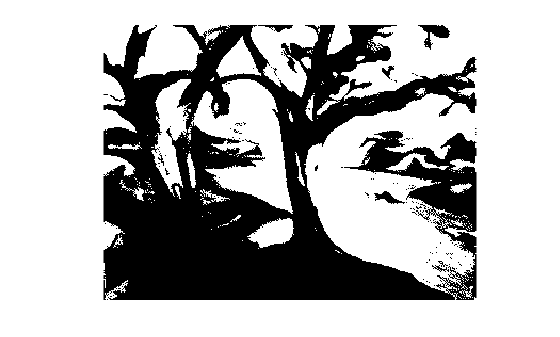# im2bw

(Not recommended) Convert image to binary image, based on threshold

`im2bw` is not recommended. Use `imbinarize` instead. For more information, see Compatibility Considerations.

## Syntax

``BW = im2bw(I,level)``
``BW = im2bw(X,cmap,level)``
``BW = im2bw(RGB,level)``

## Description

example

````BW = im2bw(I,level)` converts the grayscale image `I` to binary image `BW`, by replacing all pixels in the input image with luminance greater than `level` with the value `1` (white) and replacing all other pixels with the value `0` (black).This range is relative to the signal levels possible for the image's class. Therefore, a `level` value of `0.5` corresponds to an intensity value halfway between the minimum and maximum value of the class.```
````BW = im2bw(X,cmap,level)` converts the indexed image `X` with colormap `cmap` to a binary image.```
````BW = im2bw(RGB,level)` converts the truecolor image `RGB` to a binary image.```

## Examples

collapse all

```load trees BW = im2bw(X,map,0.4); imshow(X,map), figure, imshow(BW)```## Input Arguments

collapse all

2-D grayscale image, specified as an m-by-n numeric matrix.

Data Types: `single` | `double` | `int16` | `uint8` | `uint16`

2-D indexed image, specified as an m-by-n numeric matrix.

Data Types: `single` | `double` | `int16` | `uint8` | `uint16`

Colormap associated with indexed image `X`, specified as a c-by-3 numeric matrix with values in the range [0, 1]. Each row is a three-element RGB triplet that specifies the red, green, and blue components of a single color of the colormap.

Data Types: `single` | `double` | `int16` | `uint8` | `uint16`

2-D RGB image, specified as an m-by-n-by-3 numeric matrix.

Data Types: `single` | `double` | `int16` | `uint8` | `uint16`

Luminance threshold, specified as a number in the range [0, 1]. To compute `level`, you can use the `graythresh` function.

Data Types: `single` | `double` | `int16` | `uint8` | `uint16`

## Output Arguments

collapse all

Binary image, returned as an m-by-n logical matrix.

Data Types: `logical`

## Algorithms

If the input image is not a grayscale image, `im2bw` converts the input image to grayscale using `ind2gray` or `rgb2gray`, and then converts this grayscale image to binary by thresholding.

## Version History

Introduced before R2006a

expand all